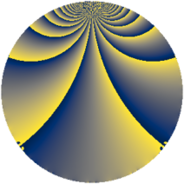# Properties

 Label 784.2.pLevel $784$ Weight $2$ Character orbit 784.p Rep. character $\chi_{784}(31,\cdot)$ Character field $\Q(\zeta_{6})$ Dimension $40$ Newform subspaces $10$ Sturm bound $224$ Trace bound $11$

# Related objects

## Defining parameters

 Level: $$N$$ $$=$$ $$784 = 2^{4} \cdot 7^{2}$$ Weight: $$k$$ $$=$$ $$2$$ Character orbit: $$[\chi]$$ $$=$$ 784.p (of order $$6$$ and degree $$2$$) Character conductor: $$\operatorname{cond}(\chi)$$ $$=$$ $$28$$ Character field: $$\Q(\zeta_{6})$$ Newform subspaces: $$10$$ Sturm bound: $$224$$ Trace bound: $$11$$ Distinguishing $$T_p$$: $$3$$, $$5$$, $$11$$

## Dimensions

The following table gives the dimensions of various subspaces of $$M_{2}(784, [\chi])$$.

Total New Old
Modular forms 272 40 232
Cusp forms 176 40 136
Eisenstein series 96 0 96

## Trace form

 $$40q - 20q^{9} + O(q^{10})$$ $$40q - 20q^{9} + 32q^{25} + 24q^{29} + 36q^{33} + 4q^{37} - 36q^{45} - 12q^{53} - 104q^{57} - 36q^{61} + 36q^{65} + 12q^{73} - 56q^{81} + 120q^{85} + 36q^{89} - 28q^{93} + O(q^{100})$$

## Decomposition of $$S_{2}^{\mathrm{new}}(784, [\chi])$$ into newform subspaces

Label Dim. $$A$$ Field CM Traces $q$-expansion
$$a_2$$ $$a_3$$ $$a_5$$ $$a_7$$
784.2.p.a $$2$$ $$6.260$$ $$\Q(\sqrt{-3})$$ None $$0$$ $$-2$$ $$-6$$ $$0$$ $$q+(-2+2\zeta_{6})q^{3}+(-4+2\zeta_{6})q^{5}+\cdots$$
784.2.p.b $$2$$ $$6.260$$ $$\Q(\sqrt{-3})$$ None $$0$$ $$-2$$ $$6$$ $$0$$ $$q+(-2+2\zeta_{6})q^{3}+(4-2\zeta_{6})q^{5}-\zeta_{6}q^{9}+\cdots$$
784.2.p.c $$2$$ $$6.260$$ $$\Q(\sqrt{-3})$$ None $$0$$ $$-1$$ $$-3$$ $$0$$ $$q+(-1+\zeta_{6})q^{3}+(-2+\zeta_{6})q^{5}+2\zeta_{6}q^{9}+\cdots$$
784.2.p.d $$2$$ $$6.260$$ $$\Q(\sqrt{-3})$$ None $$0$$ $$1$$ $$-3$$ $$0$$ $$q+(1-\zeta_{6})q^{3}+(-2+\zeta_{6})q^{5}+2\zeta_{6}q^{9}+\cdots$$
784.2.p.e $$2$$ $$6.260$$ $$\Q(\sqrt{-3})$$ None $$0$$ $$2$$ $$-6$$ $$0$$ $$q+(2-2\zeta_{6})q^{3}+(-4+2\zeta_{6})q^{5}-\zeta_{6}q^{9}+\cdots$$
784.2.p.f $$2$$ $$6.260$$ $$\Q(\sqrt{-3})$$ None $$0$$ $$2$$ $$6$$ $$0$$ $$q+(2-2\zeta_{6})q^{3}+(4-2\zeta_{6})q^{5}-\zeta_{6}q^{9}+\cdots$$
784.2.p.g $$4$$ $$6.260$$ $$\Q(\sqrt{-3}, \sqrt{7})$$ None $$0$$ $$0$$ $$6$$ $$0$$ $$q+\beta _{1}q^{3}+(2+\beta _{2})q^{5}+4\beta _{2}q^{9}+(-\beta _{1}+\cdots)q^{11}+\cdots$$
784.2.p.h $$8$$ $$6.260$$ 8.0.339738624.1 None $$0$$ $$0$$ $$0$$ $$0$$ $$q+(2\beta _{1}-\beta _{5})q^{3}+(\beta _{1}-\beta _{3})q^{5}+(-3+\cdots)q^{9}+\cdots$$
784.2.p.i $$8$$ $$6.260$$ 8.0.339738624.1 None $$0$$ $$0$$ $$0$$ $$0$$ $$q+(2\beta _{1}-\beta _{5})q^{3}+(-\beta _{1}+\beta _{3})q^{5}+(-3+\cdots)q^{9}+\cdots$$
784.2.p.j $$8$$ $$6.260$$ 8.0.339738624.1 $$\Q(\sqrt{-1})$$ $$0$$ $$0$$ $$0$$ $$0$$ $$q-\beta _{3}q^{5}+3\beta _{4}q^{9}+(\beta _{1}+\beta _{3}+\beta _{6}+\cdots)q^{13}+\cdots$$

## Decomposition of $$S_{2}^{\mathrm{old}}(784, [\chi])$$ into lower level spaces

$$S_{2}^{\mathrm{old}}(784, [\chi]) \cong$$ $$S_{2}^{\mathrm{new}}(28, [\chi])$$$$^{\oplus 6}$$$$\oplus$$$$S_{2}^{\mathrm{new}}(112, [\chi])$$$$^{\oplus 2}$$$$\oplus$$$$S_{2}^{\mathrm{new}}(196, [\chi])$$$$^{\oplus 3}$$